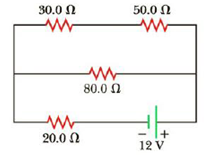Chapter 18, Problem 24P

Chapter
Section
Textbook Problem

Four resistors are connected to a battery with a terminal voltage of 12 V, as shown in Figure P18.24. (a) How would you reduce the circuit to an equivalent single resistor connected to the battery? Use this procedure to find the equivalent resistance of the circuit. (b) Find the current delivered by the battery to this equivalent resistance. (c) Determine the power delivered by the battery. (d) Determine the power delivered to the 50.0-Ω resistor.Figure P18.24

(a)

To determine
To reduce the circuits to an equivalent single resistor Req connected to the batteries.

Explanation

Given Info: Resistance R1 is 30.0Ω , Resistance R2 is 50.0Ω , Resistance R3 is 80.0Ω Resistance R4 is 20.0Ω .

Explanation:

The below figure shows the separation of loop in the circuit

Formula to calculate to reduce the circuit to an equivalent single resistor is by

Req=Rmiddle+R lower (I)

• . R upper is equivalent resistance in the upper loop
• Rlower is equivalent resistance in the lower loop
• Rmiddle is equivalent resistance in the middle loop
• R1,R2,R3,R4,R5 is corresponding resistance in the circuits
• I1,I2,I3 is corresponding current in the circuits

Consider a to b as upper loop

From the Upper loop: Resistance are connected in series

Rupper=R1+R2

Substitute 30.0Ω for R1 and 50.0Ω for R2 in the above equation to find Rupper

Rupper=30.0Ω+50.0Ω=80

(b)

To determine
The current I delivered by the battery to the equivalent resistance in the circuit.

(c)

To determine
The Power P delivered by the battery in the circuit.

(d)

To determine
The Power P50Ω delivered to the 50.0Ω resistor in the circuit.

Still sussing out bartleby?

Check out a sample textbook solution.

See a sample solution

The Solution to Your Study Problems

Bartleby provides explanations to thousands of textbook problems written by our experts, many with advanced degrees!

Get Started

Find more solutions based on key concepts